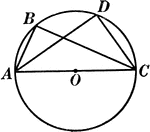### Angles Inscribed in the Same Segment Circle Proof

Illustration of a circle used to prove "All angles inscribed in the same segment are equal."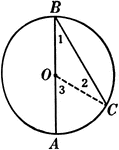### Inscribed Angle in a Circle Proof

Illustration of a circle with an inscribed angle that can be used to prove that "An inscribed angle…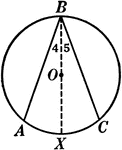### Inscribed Angle in a Circle Proof

Illustration of a circle with an inscribed angle that can be used to prove that "An inscribed angle…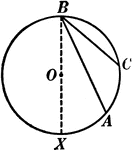### Inscribed Angle in a Circle Proof

Illustration of a circle with an inscribed angle that can be used to prove that "An inscribed angle…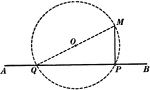### Obtuse Angles Inscribed in Circle Proof

Illustration of a circle used to prove "Any angle inscribed in a segment less than a semicircle is an…### Circle with Arc, Chord, Inscribed Angle, Circumscribed Polygon

Illustrations of a circle with arc, chord, inscribed angle, and circumscribed about a polygon.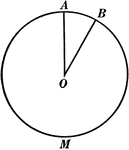### Central Angle in a Circle

Illustration of a circle with central angle AOB.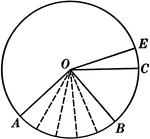### Central Angles and Arcs in a Circle

Illustration of a circle that can be used to show that an "angle at the center of a circle is measured…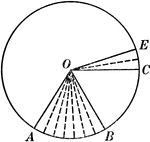### Central Angles and Arcs in a Circle

Illustration of a circle that can be used to show that an "angle at the center of a circle is measured…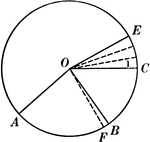### Central Angles and Arcs in a Circle

Illustration of a circle that can be used to show that an "angle at the center of a circle is measured…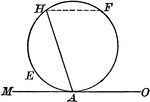### Circle With Chord and Tangent

Illustration of a circle showing an angle included by a tangent and a chord drawn from the point of…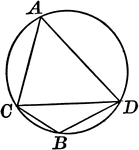### Circle With Inscribed Angle Greater Than Semicircle

Illustration of a circle with an angle inscribed in a segment greater than a semicircle, an acute angle.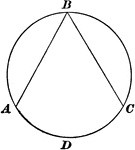### Inscribed Angle in a Circle

Illustration of a circle with an inscribed angle.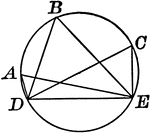### Circle With Inscribed Angle Less Than Semicircle

Illustration of a circle with an angle inscribed in a segment less than a semicircle, an obtuse angle.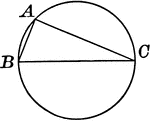### Circle With Inscribed Right Angle in Semicircle

Illustration of a circle with a right angle inscribed in a semicircle.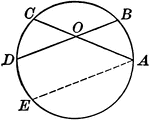### Circle With Two Intersecting Chords

Illustration of a circle with two intersecting chords within the circumference. The angle formed is…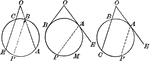### Circles With Angles Formed by Secants and Tangents

Illustration showing angles formed by two secants, two tangents, or a tangent and a secant, drawn to…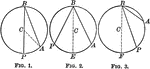### Circles With Inscribed Angles

Illustration of equal circles to show that an inscribed angle is measured by half the arc intercepted…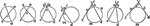### Intersecting Lines in Circles

Illustration showing various circles and the angles formed by intersecting lines.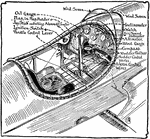### Aeroplane Cockpit

A pilot's cockpit from a propeller aeroplane, or airplane. The diagram illustrates the different parts…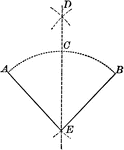### Construction of Bisecting a Given Angle

Illustration of the construction used to bisect a given angle.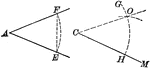### Construction of an Equal Angle Given a Point and a Straight Line

Illustration of the construction used to create an equal angle given a point in a given straight line…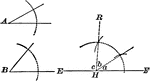### Construction to Find the Third Angle of a Triangle When Given Two Angles

Illustration of the construction used to find the third angle of a triangle when two of the angles are…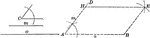### Construction of a Parallelogram When Given Two Sides and Included Angle

Illustration of the construction used to make a parallelogram when given two sides and the included…### Construction of a Triangle When Given Two Sides and the Angle Opposite (Ambiguous Case)

Illustration of the construction used to make a triangle when given two sides and the angle opposite…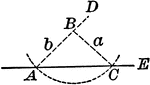### Construction of a Triangle When Given Two Sides and the Angle Opposite (Ambiguous Case)

Illustration of the construction used to make a triangle when given two sides and the angle opposite…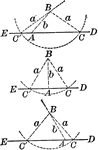### Construction of a Triangle When Given Two Sides and the Angle Opposite (Ambiguous Case)

Illustration of the construction used to make a triangle when given two sides and the angle opposite…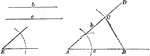### Construction of a Triangle When Given Two Sides and Included Angle

Illustration of the construction used to make a triangle when given two sides and the included angle.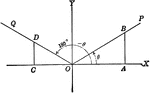### Coordinate Axis With Angles, Lines, and Perpendiculars Drawn

Coordinate axis with angle XOP equal to theta, Θ, and angle XOQ=180 - Θ. From any point in the terminal…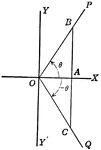### Coordinate Axis With Perpendiculars Drawn To Form Similar Right Triangles From Positive and Negative Theta, Θ

Angle XOP=Θ and angle XOQ=- Θ. From a point in the terminal side of each a perpendicular line is drawn…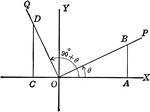### Coordinate Axis With Perpendiculars Drawn To Form Similar Right Triangles

Angle XOP=Θ and angle XOQ=90+Θ. From a point in the terminal side of each a perpendicular line is…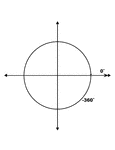### 0° and -360° Coterminal Angles

Illustration showing coterminal angles of 0° and -360°. Coterminal angles are angles drawn in…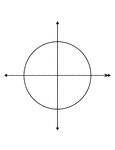### 0° and -360° Coterminal Angles

Illustration showing coterminal angles of 0° and -360°. Coterminal angles are angles drawn in…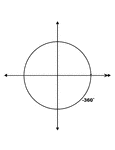### 0° and -360° Coterminal Angles

Illustration showing coterminal angles of 0° and -360°. Coterminal angles are angles drawn in…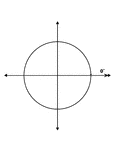### 0° and -360° Coterminal Angles

Illustration showing coterminal angles of 0° and -360°. Coterminal angles are angles drawn in…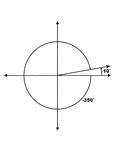### 10° and -350° Coterminal Angles

Illustration showing coterminal angles of 10° and -350°. Coterminal angles are angles drawn…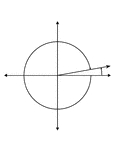### 10° and -350° Coterminal Angles

Illustration showing coterminal angles of 10° and -350°. Coterminal angles are angles drawn…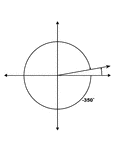### 10° and -350° Coterminal Angles

Illustration showing coterminal angles of 10° and -350°. Coterminal angles are angles drawn…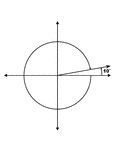### 10° and -350° Coterminal Angles

Illustration showing coterminal angles of 10° and -350°. Coterminal angles are angles drawn…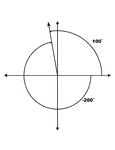### 100° and -260° Coterminal Angles

Illustration showing coterminal angles of 100° and -260°. Coterminal angles are angles drawn…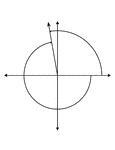### 100° and -260° Coterminal Angles

Illustration showing coterminal angles of 100° and -260°. Coterminal angles are angles drawn…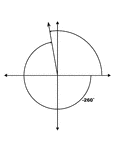### 100° and -260° Coterminal Angles

Illustration showing coterminal angles of 100° and -260°. Coterminal angles are angles drawn…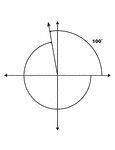### 100° and -260° Coterminal Angles

Illustration showing coterminal angles of 100° and -260°. Coterminal angles are angles drawn…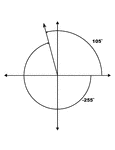### 105° and -255° Coterminal Angles

Illustration showing coterminal angles of 105° and -255°. Coterminal angles are angles drawn…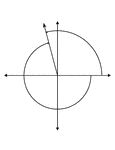### 105° and -255° Coterminal Angles

Illustration showing coterminal angles of 105° and -255°. Coterminal angles are angles drawn…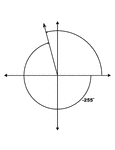### 105° and -255° Coterminal Angles

Illustration showing coterminal angles of 105° and -255°. Coterminal angles are angles drawn…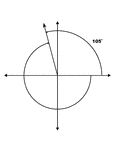### 105° and -255° Coterminal Angles

Illustration showing coterminal angles of 105° and -255°. Coterminal angles are angles drawn…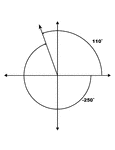### 110° and -250° Coterminal Angles

Illustration showing coterminal angles of 110° and -250°. Coterminal angles are angles drawn…### 110° and -250° Coterminal Angles

Illustration showing coterminal angles of 110° and -250°. Coterminal angles are angles drawn…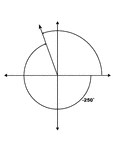### 110° and -250° Coterminal Angles

Illustration showing coterminal angles of 110° and -250°. Coterminal angles are angles drawn…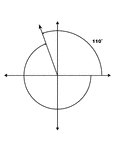### 110° and -250° Coterminal Angles

Illustration showing coterminal angles of 110° and -250°. Coterminal angles are angles drawn…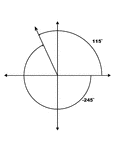### 115° and -245° Coterminal Angles

Illustration showing coterminal angles of 115° and -245°. Coterminal angles are angles drawn…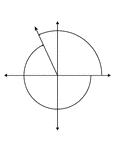### 115° and -245° Coterminal Angles

Illustration showing coterminal angles of 115° and -245°. Coterminal angles are angles drawn…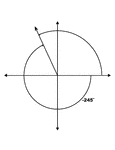### 115° and -245° Coterminal Angles

Illustration showing coterminal angles of 115° and -245°. Coterminal angles are angles drawn…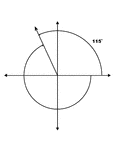### 115° and -245° Coterminal Angles

Illustration showing coterminal angles of 115° and -245°. Coterminal angles are angles drawn…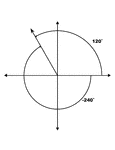### 120° and -240° Coterminal Angles

Illustration showing coterminal angles of 120° and -240°. Coterminal angles are angles drawn…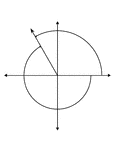### 120° and -240° Coterminal Angles

Illustration showing coterminal angles of 120° and -240°. Coterminal angles are angles drawn…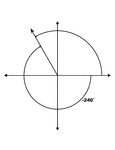### 120° and -240° Coterminal Angles

Illustration showing coterminal angles of 120° and -240°. Coterminal angles are angles drawn…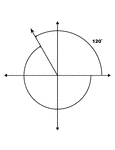### 120° and -240° Coterminal Angles

Illustration showing coterminal angles of 120° and -240°. Coterminal angles are angles drawn…### 125° and -235° Coterminal Angles

Illustration showing coterminal angles of 125° and -235°. Coterminal angles are angles drawn…